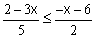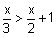Courses

# Test: Graphical Solutions

## 20 Questions MCQ Test Mathematics (Maths) Class 11 | Test: Graphical Solutions

Description
This mock test of Test: Graphical Solutions for JEE helps you for every JEE entrance exam. This contains 20 Multiple Choice Questions for JEE Test: Graphical Solutions (mcq) to study with solutions a complete question bank. The solved questions answers in this Test: Graphical Solutions quiz give you a good mix of easy questions and tough questions. JEE students definitely take this Test: Graphical Solutions exercise for a better result in the exam. You can find other Test: Graphical Solutions extra questions, long questions & short questions for JEE on EduRev as well by searching above.
QUESTION: 1

### Find the pairs of consecutive even positive integers both of which are smaller than 10 and their sum of more than 11

Solution:

Let x be the smaller of the two consecutive even positive integers .
Then the other integer is x+2.
Since both the integers are smaller than 10,x<10 ....(1)
Also the sum of the two integers is more than 11.
x+(x+2)>11
⇒ 2x+2>11
⇒ 2x>11−2
⇒ 2x>9
⇒ x>9/2
⇒ x>4.5....(2)
From (1) and (2) we obtain 4.5>x>11
Since x is an even number, x can take the values 6,8 and 10.
Thus the required possible pairs are (6,8).

QUESTION: 2

### The solution to |3x – 1| + 1 < 3 is

Solution:

|3x - 1| + 1 < 3
|3x -1| < 2
Opening mod, we get
3x - 1 < 2,  -3x + 1 > 2
3x < 3,   -3x > 1
x < 1,   x > -1/3
-1/3 < x < 1

QUESTION: 3

### Which of the following is not a linear inequality?

Solution: A is a quadratic equation not a linear equality because square of a function can't be negative
QUESTION: 4

For a student to qualify for a certain course, the average of his marks in the permitted 3 attempts must be more than 60. His first two attempts yielded only 45 and 62 marks respectively. What is the minimum score required in the third attempt to qualify?

Solution:

No of attempts = 3
Average =  (45+62+x)/3=60
x=73

QUESTION: 5

Which one of them is the solution for x, when x is integer and 12 x > 30?

Solution:

12x > 30
x > 30/12
x > 2.5
x is an integer. So, minimum value of x is 3.

QUESTION: 6

Find the value of x which satisfies 5x – 3 < 7, where x is a natural number.

Solution:
QUESTION: 7

If -5x+2<7x -4, then x is

Solution:

-5x + 2 < 7x - 4
6 < 12x
x > 1/2

QUESTION: 8

The solution to 5x-3<3x+1, when x is an integer, is

Solution:

We have 5x−3<3x+1
⇒5x−3+3<3x+1+3
⇒5x<3x+4
⇒5x−3×<3x+4−3x
⇒2x<4⇒x<2
When x is an integer the solutions of the given inequality are {.............,−4,−3,−2,−1,0,1}
Hence {x / xεZ, x<2}

QUESTION: 9

The inequations -4x+1≥0 and 3-4x<0 have the common solutions given by

Solution:

-4x+1≥0 and 3-4x<0

-4x ≥ -1 and 3 > 4x

1/4 ≥ x and 3/4 > x

{¾, ¼]

QUESTION: 10

Solve the following linear inequality for x:Solution:

(2-3x)/5 ≤ (-x-6)/2
By cross multiply we get
4-6x ≤ -5x-30
-x ≤ -34
x ≥ 34

QUESTION: 11

A point P lies in the solution region of 3x – 7 > x + 3. So the possible coordinates of P are

Solution:
QUESTION: 12

A connected planar graph having 6 vertices, 7 edges contains _____________ regions.

Solution:

By euler’s formula the relation between vertices(n), edges(q) and regions(r) is given by n-q+r=2.

QUESTION: 13

If 5x+6<2x-3, then

Solution:

5x+6<2x-3
5x-2x < -3-6
3x < -9
x<-3

QUESTION: 14

The region x > -3 lies

Solution:
QUESTION: 15

If a < b then -a ______ - b

Solution:
QUESTION: 16

The solution of inequality 4x + 3 < 5x + 7 when x is a real number is

Solution:

4x+3<5x+7
4x+3−7<5x+7−7
4x−4<5x
4x−4−4x<5x−4x
−4<x
Thus, the solution set of the given inequality is (−4,∞).

QUESTION: 17

Two less than 5 times a number is greater than the third multiple of the number. So the number must be

Solution:
QUESTION: 18

What values of x satisfy -6x>24 and x is an integer?

Solution:

- 6x < 24
=> 6x < -24
x < - 4

QUESTION: 19

The solution set of, where x is a real

Solution:

X/3-x/2 > 1
-x/6 > 1
x/6
x < -6
therefore x belongs to the range (-∞,-6)

QUESTION: 20

Find the value of x when x is a natural number and 24x< 100.

Solution:

24x < 100
⇒ x < 100/24
⇒ x < 25/6
It is evident that 1,2,3 and 4 are the only natural numbers less than 25/6,
Thus  when x is a natural number ,the solutions of the given inequality are 1,2,3 and 4.
Hence, in this case, the solution set is {1,2,3,4}.